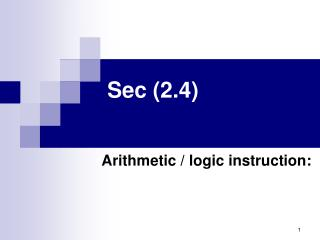DownloadDownload PresentationSec (2.4)

# Sec (2.4)

Download Presentation## Sec (2.4)

- - - - - - - - - - - - - - - - - - - - - - - - - - - E N D - - - - - - - - - - - - - - - - - - - - - - - - - - -
##### Presentation Transcript

1. Sec (2.4) Arithmetic / logic instruction:

2. Logical operations: • Ex:

3. Mask: • Suppose we have this byte 00110011 we need to find a mask that being given the inverse for all digits? • Sol.: 00110011 XOR 11111111 11001100

4. Mask: So, note that: • X AND 0 0 • X AND 1 X • X OR 0 X • X OR 1 1 • X XOR 0 X • X XOR 1 X

5. Rotation and shift operations: • The operation in the class of rotation and shift operations provide a means for moving bits within a register and are often used in solving alignment problems. These operation are classified by the direction of motion (right or left) and whether the process is circular. Within these classification guidelines are numerous variations with mixed terminology. • circular shift (rotation) • logical shift • arithmetic shift

6. circular shift (rotation) • Ex: Rotating the bit pattern A3 one bit to the right

7. logical shift: • another technique is to discard the bit that falls of the edge and always fill the hole with a 0. • Ex: +437 / 8 = 437 / 23 it means that shift 3 bits to right

8. logical shift:

9. arithmetic shift: • in either shift, care must be taken to preserve the sign bit when using certain notational system • we often find right shifts that always fill the hole with its original value • shifts that leave the sign bit unchanged • Ex: +50 * 16 = 50 * 24 it means that shift 4 bits to left

10. arithmetic shift: